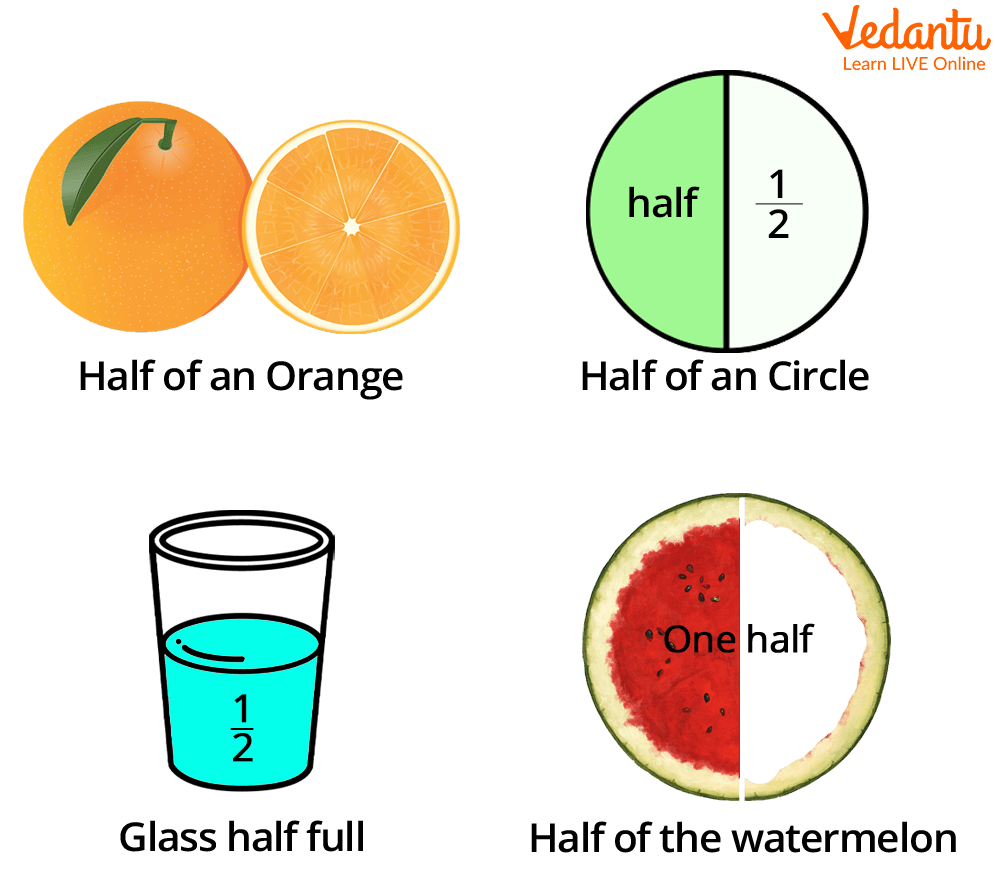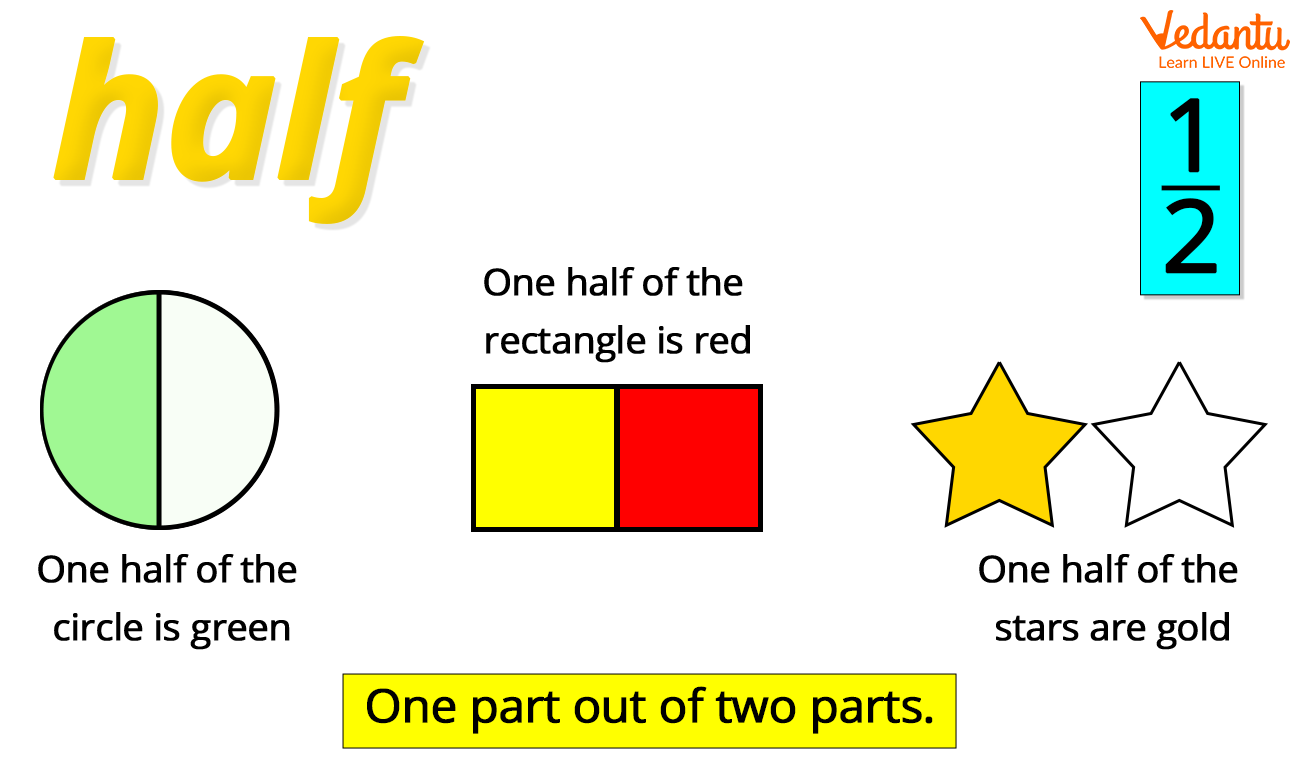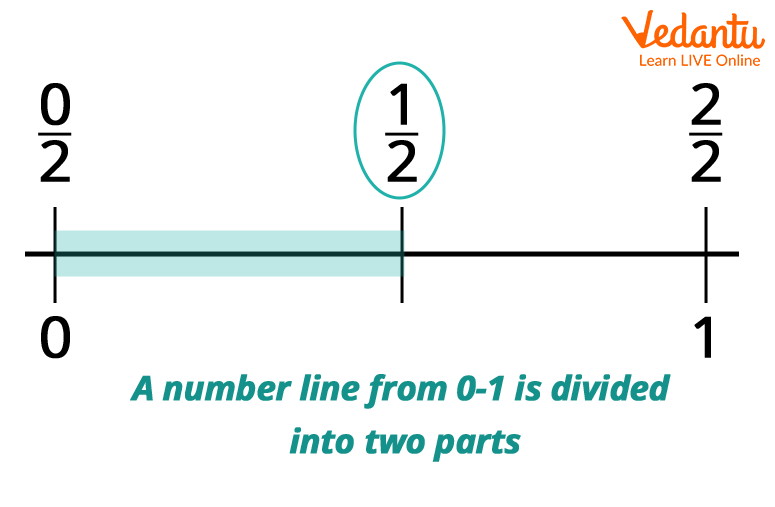Courses
Courses for Kids
Free study material
Free LIVE classes
More

# Half Numbers: Representation of Half on a Number Line## What are Halves?

While dividing things into two equal parts, we often say that it is now divided into two halves. So splitting a whole thing or object into two equal parts gives two halves of the particular thing. We come across several examples of halves in our daily lives. For example, we can split an orange, or watermelon into halves, a circle into halves, and so on. Therefore, to get half of a number we need to divide it into two equal parts by dividing it by 2.

Let’s read the following sections to know more about a half number in detail.Examples of Halves in our daily life

## What is $\frac{1}{2}$?

We know that when a whole thing is divided into two equal parts, each part obtained is called a half. Similarly, numbers can also be divided into two halves. When we divide the number 1, we obtain two$\frac{1}{2}$’s. So $\frac{1}{2}$ means a whole number which is 1 divided by 2. $\frac{1}{2}$ is the representation of half in fraction form.

A fraction is a part of a whole thing. For example, when you take a slice of cake, it becomes a fraction (a piece) of the entire cake. Similarly, half is one of the two parts.Examples of one out of two parts

## Representing Half on a Number Line

The number line is a visual representation of numbers on a line. We can also represent half on a number line. The distance between 0 and 1 on a number line is always one, i.e., the whole number. So when we divide this distance into two equal parts we will get two halves. The representation of half on a number line is given below.Representing half on a number line

## Properties of Halves

A few properties of halves are given below.

• Half is written as 0.5 in decimal form.

• You can calculate half of a number by multiplying it by $\frac{1}{2}$. For example, half of the number 8 can be obtained by multiplying 8 by $\frac{1}{2}$ i.e., 8 x$\frac{1}{2}$=4.

• Half is always two equal parts of one whole thing.

• The addition of two halves makes one.

## Conclusion

The above article provides all the necessary and relevant information that a child needs to know about a half number. The above information thus covered the representation of half on a number line and a few properties of halves.

Last updated date: 21st Sep 2023
Total views: 131.7k
Views today: 1.31k

## FAQs on Half Numbers: Representation of Half on a Number Line

1. How can you calculate half of any given number?

We know that half is an equal part of a whole. Hence when we need to divide anything into halves we need to divide that into two equal parts. So half of any given number can be calculated by dividing the number by 2 or by multiplying the number by $\frac{1}{2}$. For example, half of 6 can be calculated by dividing it by 2 i.e., 6/2 = 3 or by multiplying it by $\frac{1}{2}$, i.e., 6 x$\frac{1}{2}$ = 3.

2. How many halves are there in a whole?

There will always be two halves in a whole. Two halves make a whole thing.